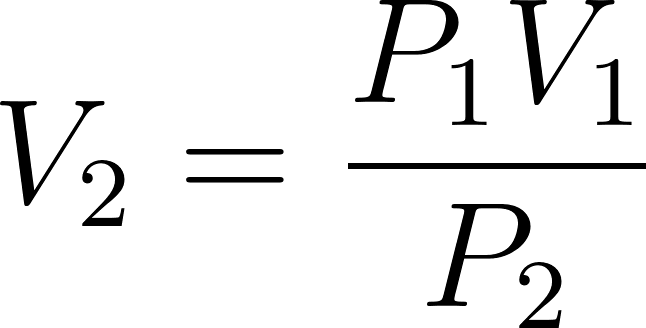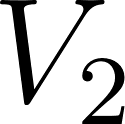×
Get Full Access to Introductory Chemistry - 5 Edition - Chapter 11 - Problem 35p
Get Full Access to Introductory Chemistry - 5 Edition - Chapter 11 - Problem 35p

×

# Solved: A snorkeler with a lung capacity of 6.3 L inhales a lungful of air at theISBN: 9780321910295 34

## Solution for problem 35P Chapter 11

Introductory Chemistry | 5th Edition

• Textbook Solutions
• 2901 Step-by-step solutions solved by professors and subject experts
• Get 24/7 help from StudySoup virtual teaching assistantsIntroductory Chemistry | 5th Edition

4 5 1 257 Reviews
21
4
Problem 35P

PROBLEM 35P

A snorkeler with a lung capacity of 6.3 L inhales a lungful of air at the surface, where the pressure is 1.0 atm. The snorkeler then descends to a depth of 25 m, where the pressure increases to 3.5 atm. What is the capacity of the snorkeler's lungs at this depth? (Assume constant temperature.)

Step-by-Step Solution:

Problem 35P

A snorkeler with a lung capacity of 6.3 L inhales a lungful of air at the surface, where the pressure is 1.0 atm. The snorkeler then descends to a depth of 25 m, where the pressure increases to 3.5 atm. What is the capacity of the snorkeler's lungs at this depth? (Assume constant temperature.)

Step by Step Solution

Step 1 of 2

According to Boyle's law, at constant temperature, pressure is inversely proportional to its volume of a certain gas................(1)

Rearrange the formula (1)...........(2)= initial pressure of the gas= Initial volume of the gas= Final pressure of the gas= final volume of the gas.

Step 2 of 2

##### ISBN: 9780321910295

Unlock Textbook Solution